Mike on MATLAB GraphicsGraphics & Data Visualization

Note

Mike on MATLAB Graphics has been retired and will not be updated.

Tiling Hexagons and Other Permutohedra

In earlier posts we've looked at tiling quadrilaterals and pentagons. So what about hexagons? I'm sure you've seen tilings of regular hexagons. They're popular in floor tiles and game tiles. Even bees know about this one. But there's one interesting feature of this tiling that you might not be familiar with. To see this feature, we need to start in a very different place.

We're going to start by making all of the permutations of the sequence [1 2 3]. As you probably know, for n items, there are n! permutations. So there are 6 different ways we can arrange these 3 numbers.

n = 3;
np = factorial(n);
pts = perms(1:n)
pts =

3     2     1
3     1     2
2     3     1
2     1     3
1     2     3
1     3     2

Now we have an array with 6 rows and 3 columns. Since we have 3 columns, I'm just going to go ahead and use them as coordinates in 3D space. That gives me 6 points that I can draw with the stem3 function.

stem3(pts(:,1),pts(:,2),pts(:,3),'filled')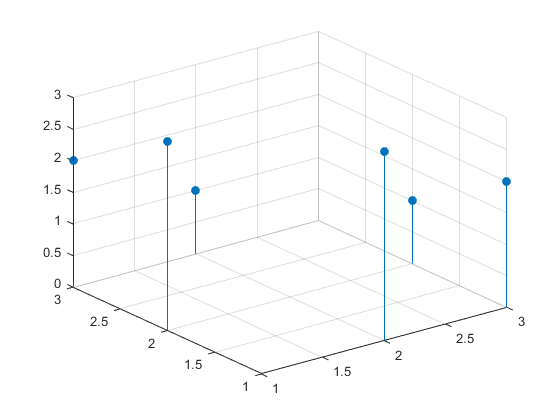These points are all arranged on the edges of a box that goes from 1 to 3 along each axis.

lx = [1 3 3 1 1 nan 1 3 3 1 1 nan 1 1 nan 3 3 nan 3 3 nan 1 1 nan];
ly = [1 1 3 3 1 nan 1 1 3 3 1 nan 1 1 nan 1 1 nan 3 3 nan 3 3 nan];
lz = [1 1 1 1 1 nan 3 3 3 3 3 nan 1 3 nan 1 3 nan 1 3 nan 1 3 nan];
line(lx,ly,lz,'Color',[.75 .75 .75])
xlim([0.5 3.5])
ylim([0.5 3.5])
zlim([0.5 3.5])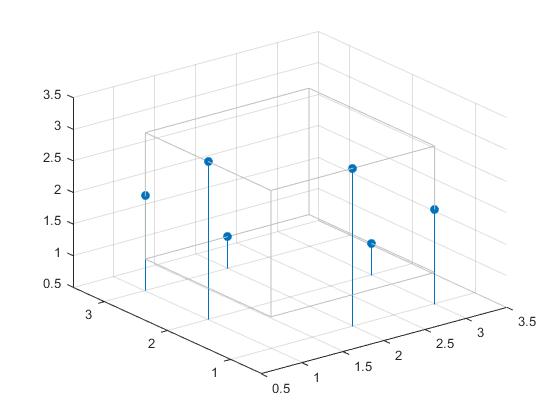Let's add some labels so we can see what we've got.

xlabel X
ylabel Y
zlabel Z
nodelabels = cellstr(arrayfun(@(x)sprintf('%d',x),pts));
for i=1:np
text(pts(i,1),pts(i,2),pts(i,3)+.1,nodelabels{i,1}, ...
'HorizontalAlignment','center', ...
'VerticalAlignment','baseline', ...
'FontSize',16);
end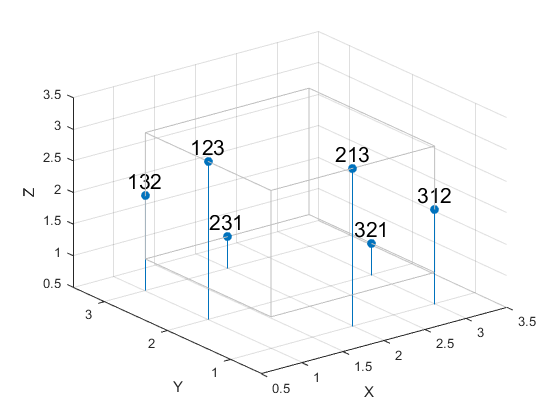Now I want to connect each pair of points that are on the same face of the cube. One way to do that is like this:

edges = [];
for i=1:np
for j=(i+1):np
diff = pts(j,:) - pts(i,:);
if isscalar(find(diff==-1)) && isscalar(find(diff==1)) && (n-2)==length(find(diff==0))
edges(end+1,:) = [i j];
end
end
end
g = graph(edges(:,1),edges(:,2));
o = dfsearch(g,1);
f = [o; 1];
line(pts(f,1),pts(f,2),pts(f,3))
f = o';You can probably already see that these form a hexagon. Let's emphasize that by creating a patch from them, and rotating around to get a better view.

cla
cols = lines(7);
patch('Faces',f,'Vertices',pts,'FaceColor',cols(1,:))
view([120 30])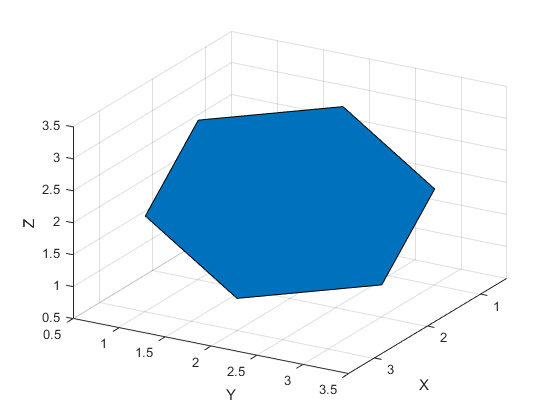And if we stacked an infinite number of boxes and intersected them all with that same plane, we would get a number of different hexagons, tiled in the familiar pattern.

cla
vec1 = pts(f(5),:) - pts(f(3),:);
vec2 = pts(f(5),:) - pts(f(1),:);
p = gobjects(0);
for i=-4:4
for j=-4:4
cindex = 1+mod(2*mod(i,2) + 1*mod(j,2),7);
tmp_pts = pts + repmat(i*vec1,[np 1]) + repmat(j*vec2,[np 1]);
p(end+1) = patch('Faces',f,'Vertices',tmp_pts,'FaceColor',cols(cindex,:));
end
end
xlim([-2 6])
ylim([-2 6])
zlim([-2 6])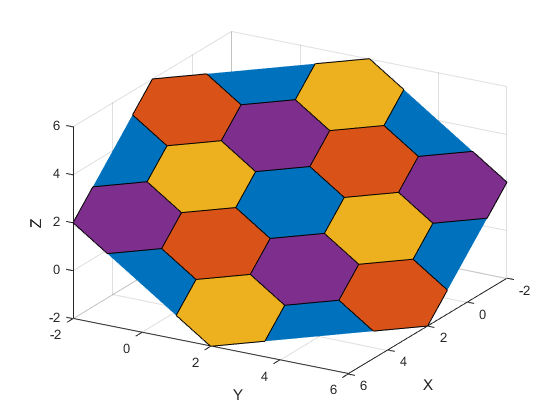And because all of these patches line in a plane, we can project them into 2D using a pair of basis vectors which are tangent to the plane.

uvec = [1, 0, 0];
vvec = [0, sqrt(2)/2, -sqrt(2)/2];

for i=1:numel(p)
v3 = p(i).Vertices;
x = sum(v3 .* repmat(uvec,[np 1]),2);
y = sum(v3 .* repmat(vvec,[np 1]),2);
p(i).Vertices = [x, y, zeros(np,1)];
end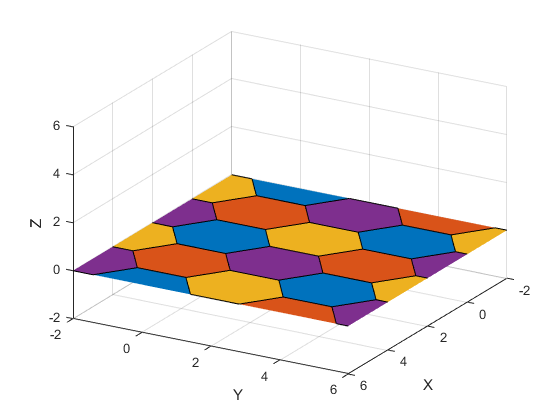And now we have the familiar 2D tiling of regular hexagons.

view(2)
axis off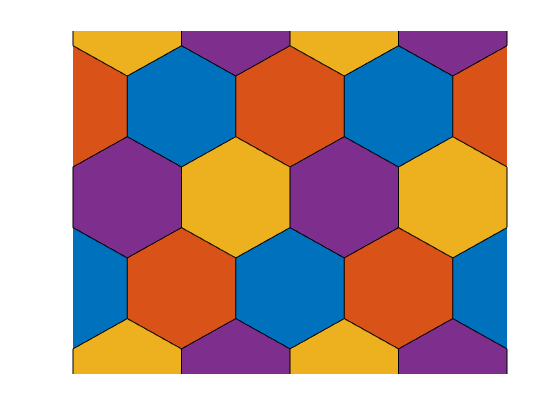That's kind of an odd way to think about hexagonal tilings, isn't it? But it's actually useful because of one surprising fact. And that is that this trick works in any dimension!

If we did this in 2D, we'd get a line connecting the points [1 2] and [2 1]. What if we did it in 4D? Let's give it a try, and see what we get.

This time we'll get an array that is 24x4 because 4! is 24.

n = 4;
np = factorial(n);
pts = perms(1:n)
pts =

4     3     2     1
4     3     1     2
4     2     3     1
4     2     1     3
4     1     2     3
4     1     3     2
3     4     2     1
3     4     1     2
3     2     4     1
3     2     1     4
3     1     2     4
3     1     4     2
2     3     4     1
2     3     1     4
2     4     3     1
2     4     1     3
2     1     4     3
2     1     3     4
1     3     2     4
1     3     4     2
1     2     3     4
1     2     4     3
1     4     2     3
1     4     3     2

If we use each row as a point, we have 4 dimensions. This makes things a bit more abstract. Let's start by drawing the points and their connections as an undirected graph.

nodelabels = cellstr(arrayfun(@(x)sprintf('%d',x),pts));
edges = [];
for i=1:np
for j=(i+1):np
diff = pts(j,:) - pts(i,:);
if isscalar(find(diff==-1)) && isscalar(find(diff==1)) && (n-2)==length(find(diff==0))
edges(end+1,:) = [i j];
end
end
end
g = graph(edges(:,1),edges(:,2),[],nodelabels);
plot(g)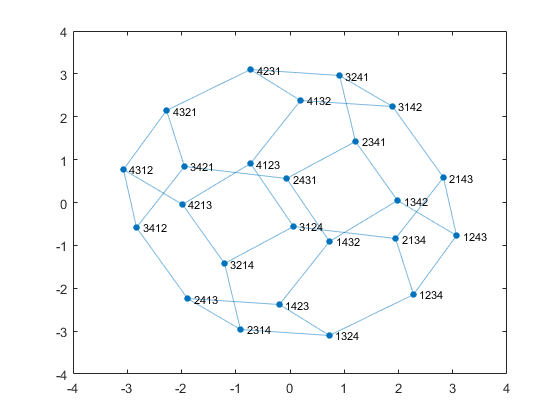We can see that there appears to be a polyhedron hiding in there.

What if we project this shape down into 3 dimensions? To do this, we'll need three 4D vectors which are normal to each other, and normal to the hyperplane. I happen to have a set right here.

u = [sqrt(2)/2, -sqrt(2)/2, 0, 0];
v = [sqrt(6)/6, sqrt(6)/6, -sqrt(2/3), 0];
w = [sqrt(12)/12, sqrt(12)/12, sqrt(12)/12, -sqrt(3)/2];

Now we can use the same technique I used to project that plane of hexagons down from 3D to 2D.

x = sum(pts .* repmat(u,[np 1]),2);
y = sum(pts .* repmat(v,[np 1]),2);
z = sum(pts .* repmat(w,[np 1]),2);
scatter3(x,y,z,'filled');
axis equal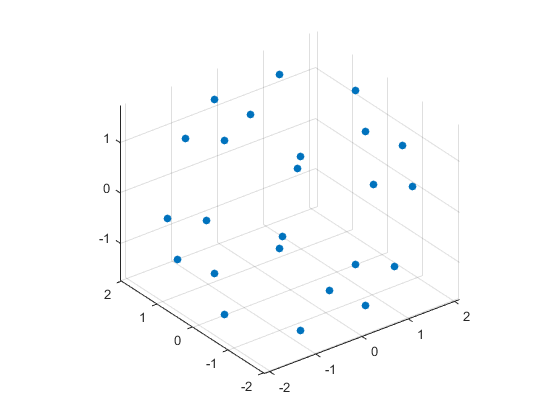And then we can get the faces of the shape by using convhull.

faces = convhull(x,y,z);

hold on
trisurf(faces,x,y,z,'FaceColor',cols(3,:),'EdgeColor','none')
axis vis3d
camlight right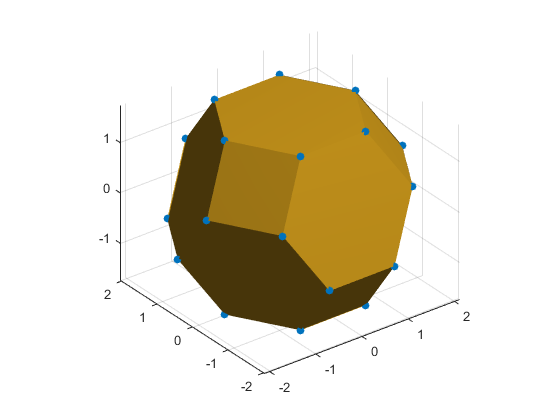And we can recognize this as a truncated octahedron.

And just as we saw in the 2D case, these octahedra will tile 3D space with no gaps.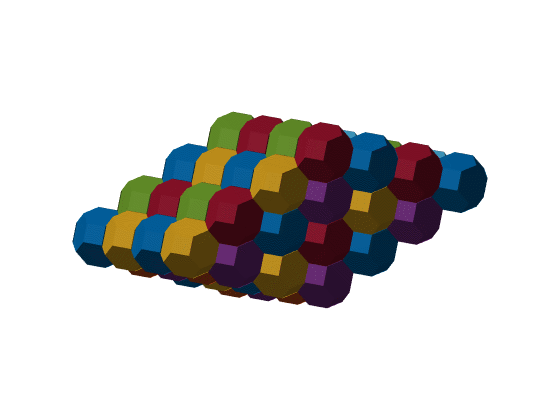This tiling is known as the bitruncated cubic honeycomb.

And as I said, you can do this same thing in any dimension to generate a set of objects which tile the next dimension down. For example, if you do this with n=5, you'll get a 4D shape called the great prismatodecachoron. This family of shapes go by the curious name of permutohedron. Aren't they an interesting family?

Published with MATLAB® R2015b